24 More Forms of Visualization
Weve seen how to plot lists of data with ListPlot and ListLinePlot. If you want to plot several sets of data at the same time, you just have to give them in a list.
 In:=Out=The PlotStyle option lets you specify the style for each set of data:
 In:=Out=The Mesh option lets you show the actual data points too:
 In:=Out=In:=Out=The histogram shows how often each length occurs among the first 200 words:
 In:=Out=In:=Out=In:=Out=Show it without a mesh:
 In:=Out=Make a contour plot, in which successive ranges of heights are separated by contour lines:
 In:=Out=Make a relief plot of the topography 100 miles around Mount Everest:
 In:=Out=ListLinePlot[{list1,list2,...}] plot several lists together Histogram[list] make a histogram ListPlot3D[array] plot an array of heights in 3D ListContourPlot[array] plot contours for an array of heights ReliefPlot[array] make a relief plot GeoElevationData[region] array of geo elevations for a region PlotStyle option for styling each set of data Mesh whether to have a mesh of points or lines MeshStyle option for styling a mesh
24.1Make a plot with lines joining the squares, the cubes and the 4th powers of integers up to 10. »
Expected output:
 Out[]=Answer & check your solution
24.2Make a plot of the first 20 primes, joined by a line, filled to the axis and with a red dot at each prime. »
Expected output:
 Out[]=Answer & check your solution
Expected output:
 Out[]=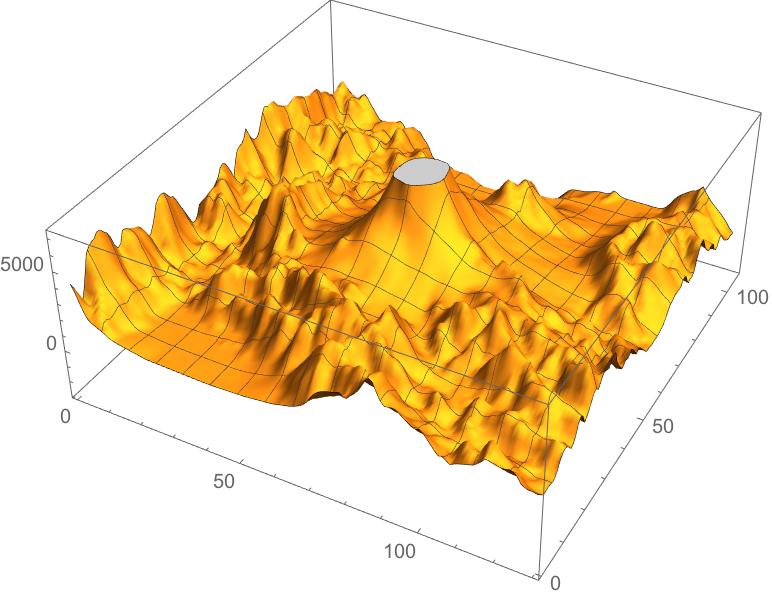Answer & check your solution
24.4Make a relief plot of the topography for 100 miles around Mount Fuji. »
Expected output:
 Out[]=Answer & check your solution
24.5Make a 3D plot of heights generated from Mod[i, j] with i and j going up to 100. »
Expected output:
 Out[]=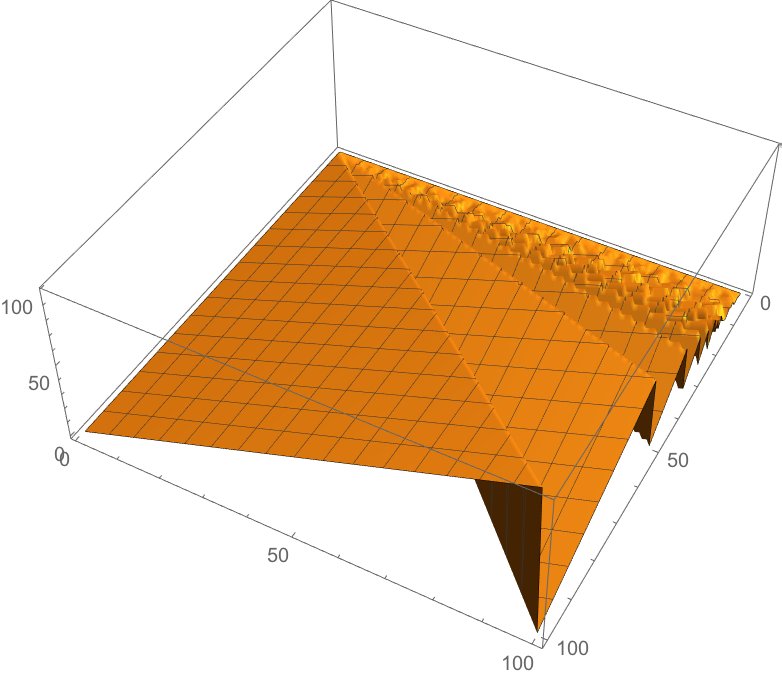Answer & check your solution
24.6Make a histogram of the differences between successive primes for the first 10000 primes. »
Expected output:
 Out[]=Answer & check your solution
Expected output:
 Out[]=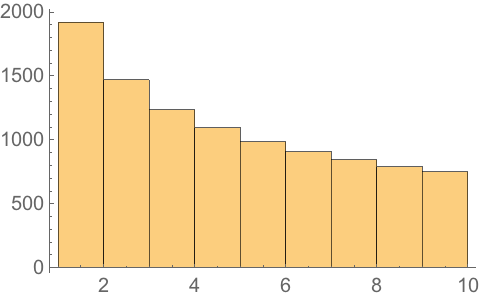Answer & check your solution
Expected output:
 Out[]=Answer & check your solution
Sample expected output:
 Out[]=Answer & check your solution
Sample expected output:
 Out[]=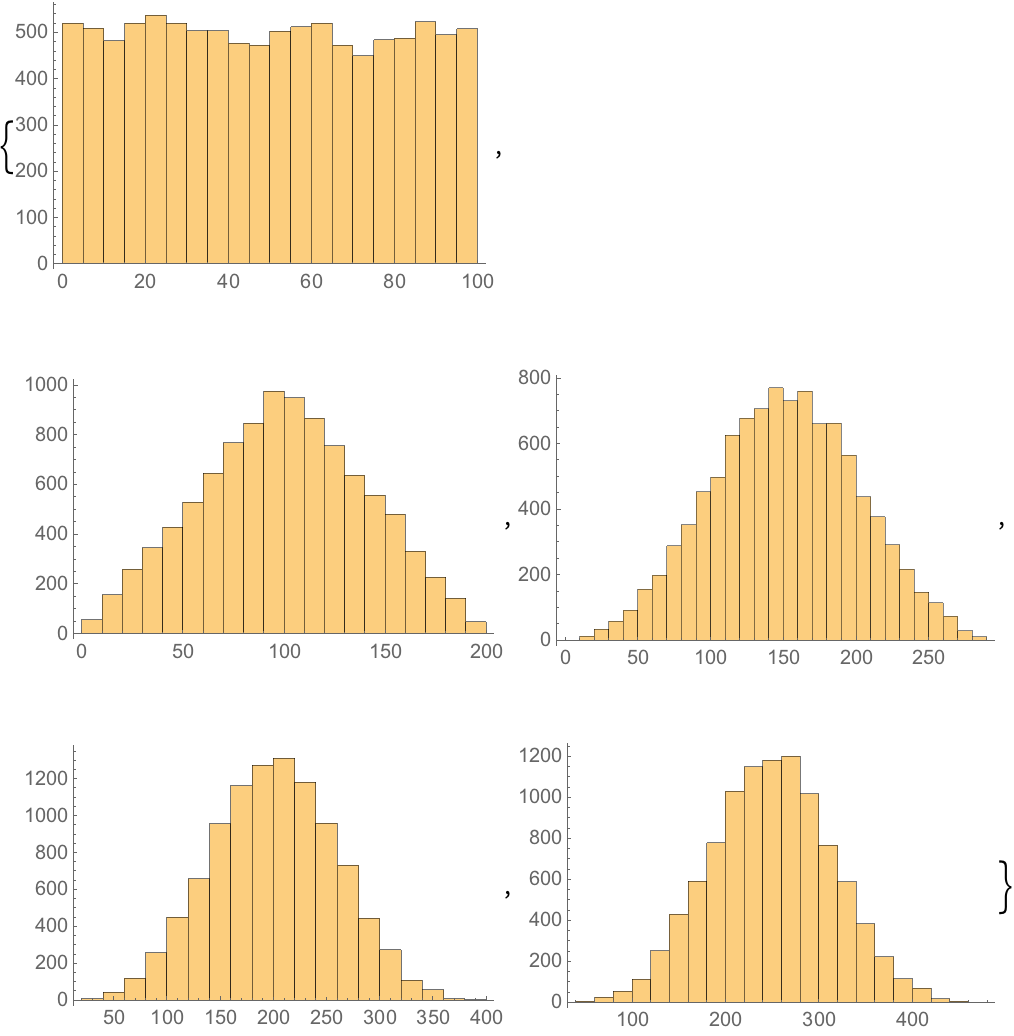Answer & check your solution
24.11Generate a 3D list plot using the image data from a binarized size-200 letter W as heights. »
Expected output:
 Out[]=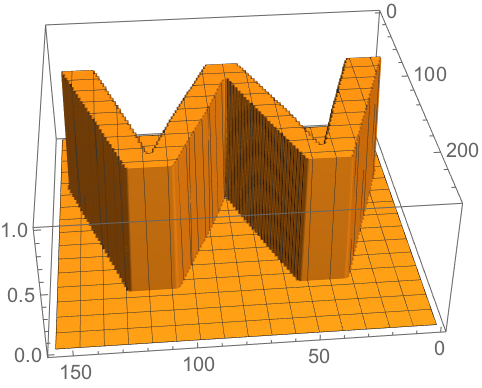Answer & check your solution
+24.1Make a histogram of word lengths in the Wikipedia article on computers. »
Sample expected output:
 Out[]=Answer & check your solution
What other kinds of visualizations are there?
How do I combine plots Ive generated separately?
Use Show to combine them on common axes. Use GraphicsGrid, etc. (see Section 37) to put them side by side.
How can I specify the bins to use in a histogram?
Histogram[list, n] uses n bins. Histogram[list, {xmin, xmax, dx}] uses bins from xmin to xmax in steps dx.
Whats the difference between a bar chart and a histogram?
How can I draw contour lines on a 3D topography plot?
Use MeshFunctions(#3&). The (#3&) is a pure function (see Section 26) that uses the third (z) coordinate to make a mesh.